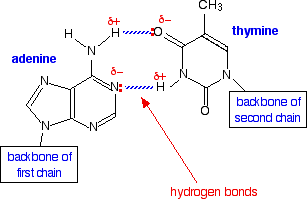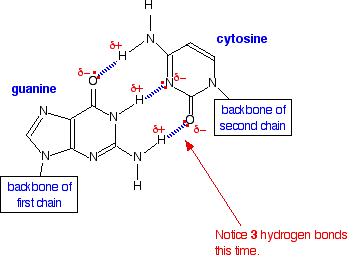# 11.5: Hydrogen Bonds

## Introduction

Figure $$\PageIndex{1}$$: In this rotating model oxygen are red, carbon grey and hydrogen white.

Hydrogen bonds are a strong type of dipole-dipole interaction. As a Rule of Thumb, they are weaker than covalent and ionic ("intramolecular") bonds", but stronger than most dipole-dipole interactions. There are two requirements for hydrogen bonding.

Two Requirements for Hydrogen Bonding:

1. First molecules has hydrogen attached to a highly electronegative atom (N,O,F).
• (hydrogen bond donor)
2. Second molecule has a lone pair of electrons on a small highly electronegative atom (N,O,F).
• (hydrogen bond acceptor)

Highly electronegative atoms like N,O,F can not completely remove the valence electron from hydrogen and form an ion because there are no core electrons in hydrogen. Removing the hydrogen's 1s electron would produce a subatomic particle, the proton, whose small size results in a high charge density that would pull back the electron. So it will not happen. The result is that hydrogen forms polar covalent bonds when attached to an electronegative atom and does not form ions. The electronegative atoms pull on the valence electron "deshields" the hydrogen's proton resulting in a large δ+ charge over a small area. A highly electronegative atom has a large δ- charge and if it has a lone pair of electrons, they are strongly attracted to the "deshielded proton" of another hydrogen and create a hydrogen bond. It should also be noted that the small size of the hydrogen allows it to move in real close, resulting is a strong bonding interaction.

Exercise $$\PageIndex{1}$$

How many hydrogens in figure $$\PageIndex{1}$$ can form hydrogen bonds?

Only one, the one at the very top which is attached to the highly electrongative oxygen atom (red), all the others are attached to carbon and can not hydrogen bond.

Does Chlorine form Hydrogen Bonds?

Even though chlorine is highly electronegative, the best answer is no, and in this class we will consider chlorine not to form hydrogen bonds (even though it has the same electronegativity as oxygen). This is because chlorine is large and its lone electron is in a diffuse orbital, covering a large area, and thus do not have the high charge density to act as a strong hydrogen bond acceptor. But it does form weak hydrogen bonds in solid crystalline hydrogen chloride at very low temperatures.

## H-Bonds and Water

H-bonding occurs in water. In the liquid state they are rapidly being formed and broken as the mobile particles move over each other. Note in figure $$\PageIndex{2}$$ that there are two type of O-H bonds, the intramolecular O-H bond within a molecule (bond length = 1.01Å) and the intermolecular bond between atoms (bond length = 1.75Å). The closeness of the bond length indicates that the intramolecular bond is very strong, and of comparable magnitude to the intramolecular one. On a macroscopic scale this is obvious to anyone who falls on ice (frozen water) and quickly realizes how hard it is, and how strong the bonds are that hold the water molecules to each other.Figure $$\PageIndex{2}$$: Hydrogen bonding interactions within water. Note the similarity in length between the intermolecular O---H bond, and the intramolecular O-H bond.

Water also has two lone pairs and two H atoms attached to the highly electronegative oxygen. This means each water molecule can participate in up to 4 bonds (two where it is the h-bond acceptor, and two where it is the h-bond donor). One interesting consequence of this is that water forms a 3D crystalline structure that is sort of based on a distorted tetrahedron. That is, the oxygen is sp3 hybridized with a tetrahedral electronic geometry, having two bonding orbitals and two lone pairs. All of these are involved with hydrogen bonds. The lone pairs are functioning as H-bond acceptors, and the hydrogen on the bonding orbitals are functioning as h-bond donors. So each oxygen is attached to 4 hydrogens, two are 1.01Å covalent bonds and two are 1.75 Å hydrogen bonds, and this results in a structure like figure 11.5.3, which has lots of void space, and the consequence that ice is less dense than liquid water and floats.Figure $$\PageIndex{3}$$: Hydrogen bonding and the crystal structure of ice (left), which floats on liquid water (rights, wikicommons image credit)

The fact that ice floats has great ramifications for life. The ice, with it's void space, acts as an insulator. If the ice sunk to the bottom, lakes would completely freeze and aquatic life like fish would not be able to survive the winters.

### H-bonding and Boiling Points

Boiling points are an indicator of intermolecular forces, and we will look at the phenomena of boiling in more detail in a later section of this chapter. From the kinetic molecular theory of gases we learned that the kinetic energy of a molecule is proportional to its absolute temperature, where KETranslation = 1/2mv2, (m is the mass, and v is the velocity). We also learned that there is a velocity profile with different molecules moving at different speeds, but that heavier molecules tend to move slower than lighter ones (remember macroscopic observables like liquids and boiling are the result of the interactions of a huge number of molecules which possess a distribution of energies). So all things equal, we would anticipate that it is easier to boil a lighter molecule than a heavier one, and we would predict the heavier one to have a higher boiling point.

Figure $$\PageIndex{4}$$. shows the trend for various hydrides of different families of the periodic table. Our predictions based on molar mass work for group IV (CH4 through SnH4), but does not work for groups VII, VI and V, where the hydrides of the second period (N,O,F) have higher boiling points than would be predicted from their mass trends.Figure $$\PageIndex{4}$$: Periodic trends in boiling point for various hydrides.

There are a multitude of questions that can come out of figure $$\PageIndex{4}$$, and you should familiarize yourself with this figure. You should also revisit this figure after we have covered the section on boiling. Right now let is suffice that to boil a substance you need to overcome the intermolecular forces that hold the liquid together (the cohesive forces), and that as you increase the temperature you increase the kinetic energy of the molecules (so they can escape the liquid and become a vapor).

First, lets look at the group 4 hydrides (CH4, SiH4, GeH4& SnH4),. As we go down the table from carbon to silicon to germanium to Tin we see an increase in the boiling points. These are symmetric nonpolar molecules and there are two reasons why going down this family shows an increase in the boiling point.

1. Going down group 4 the mass increases, requiring a higher temperature for molecules to gain enough kinetic energy to vaporize.
2. Going down the table the valence electrons occupy more diffuse orbitals, resulting in a higher polarizability, which in turn results in larger London dispersion forces, which requires more

So both of these trends would indicate an increase in the boiling point as we go down the family.

Second, ammonia, hydrofluoric acid and water show a deviation in this trend. That is, they are lighter, but there is an increase in their boiling points. This is due to hydrogen bonding. That is, although these molecules are lighter, they have very strong intermolecular forces, which must be overcome for them to boil.

Third, water has a higher boiling point than HF, yet fluorine is more electronegative than oxygen, it is also smaller, and so you would expect the HF hydrogen bond to be stronger than the OH hydrogen bond. In fact, this is all try, and what this argument does not take into account is the number of hydrogen bonds. That is, the cohesive forces that hold the liquid together are not just the strength of the bonds, but also the number of bonds. Each HF molecule has one H, and 3 lone pairs on the fluorine. So in a macroscopic system like a mole of HF (remember that to be a liquid, you need a lot of molecules), each HF would on average be involved with 2 bonds, one involving the hydrogen (hydrogen donor), and one involving a fluorine lone pair (hydrogen acceptor), and simple speaking, there are not enough hydrogens to use up all the lone pairs, (two of the fluorine's lone pairs are not involved in H bonds.) In the case of water, the number of lone pairs equals the number of hydrogens, and so each water molecule can on the average, be involved with 4 hydrogen bonds. So even though the individual hydrogen bonds in water may be weaker than in HF, there are more of them, making the boiling point of water higher than HF.

#### Exercise 11.5.2: Look at Real Molecules (Exam Material)

Before reading on, look at the following three molecules and their boiling points, and try and answer the following 2 questions.1. Why is pentane's boiling point so much lower than the other two?

Pentane is non polar and the other two have hydrogen bonds through the OH group.

2. Why does butan-1-ol have a higher boiling point than 2-methylpropan-1-ol?

Both of these molecules are isomers with the same chemical constituents (C4H10O) and both have an OH group than can be involved with hydrogen bonds. But butan-1-ol is more diffuse, thus more polarizable and has stronger van der Waals (London dispersion) interactions

## Hydrogen Bonding and Biology

We have all heard of DNA, which consists of nucleotide strands that join together to form the infamous double helix. DNA helix showing the base pairs adenine/thymine (A-T) an Guanine/Cytosine. (G-C), which form the double helix through the base pair interactions that are made through hydrogen bonding (11.5.4).Figure $$\PageIndex{5}$$: The adenine-thymine base pair held together by two hydrogen bonds(left) and the guanine-cytosine base pair held together by three hydrogen bonds (right). These are the

Proteins are based on amino acids and have 4 structural levels (Figure 11.5.5)Figure $$\PageIndex{6}$$: The 4 structural levels of proteins

The secondary level structure of proteins form alpha helixes and beta-pleated sheets, which are held together by hydrogen bonds.Figure $$\PageIndex{7}$$: Protein alpha helixes (left) and beta pleated sheets (right) maintain their secondary structure through hydrogen bonding interactions (dotted red lines in the beta pleated sheet).